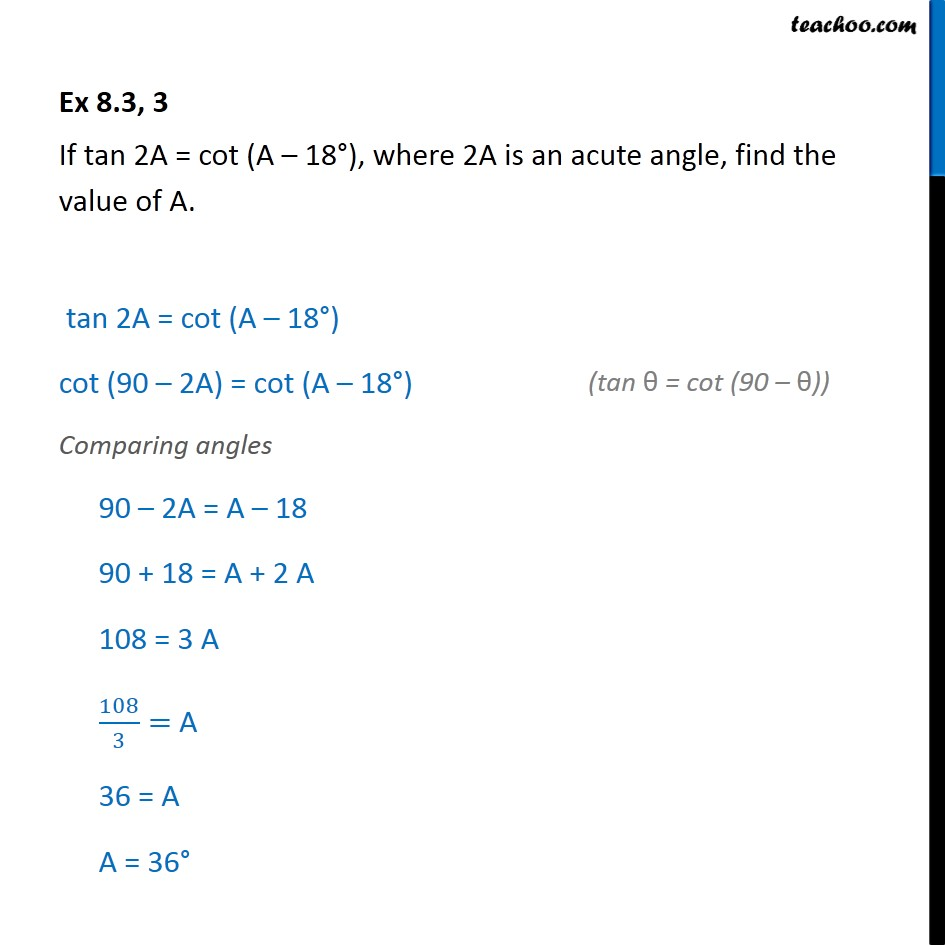Subscribe to our Youtube Channel - https://you.tube/teachoo

1. Chapter 8 Class 10 Introduction to Trignometry
2. Serial order wise
3. Ex 8.3

Transcript

Ex 8.3, 3 If tan 2A = cot (A – 18°), where 2A is an acute angle, find the value of A. tan 2A = cot (A – 18°) cot (90 – 2A) = cot (A – 18°) Comparing angles 90 – 2A = A – 18 90 + 18 = A + 2 A 108 = 3 A 108/3= A 36 = A A = 36°

Ex 8.3